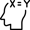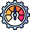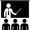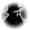# How to use Kelly Criterion in sports betting

The Kelly Criterion is a mathematical formula that can help you determine the ideal stake on a wager. The main idea is to ensure that your overall losses will be smaller than your potential profit. If you want to follow a safer path in sports betting, this math equation can secure a higher chance of winning. Τhe ultimate target is to protect your bankroll from long-term damage and grow your starting investment. The result of the mathematical formula can drive you an edge over the bookies, learning the true probabilities of your bets to succeed. Firstly, you should decide the size of your bankroll and the period of time you will use the Kelly Criterion formula for sports betting. Then, the math process will suggest the percentage of the investment you must bet.

## What is the Kelly CriterionKelly Criterion is one of the primary betting systems that can calculate the optimal stake in sports games with fixed odds. As it happens the most times in betting, that stake sizing pattern works better on single bets. For example, contrary to the Martingale system, the ultimate goal is to maximise the expected bankroll growth over a set number of wagers. That means that you will have several bets open simultaneously.

Following the Kelly formula, you can find the appropriate stake to bet on Liverpool to win at Milan for the Champions League at 3.00 odds. If you have a 40% probability of winning, the losing probability is 60%, and the result of the equation is 0.1. That means you should be staking the 10% per cent of your bankroll (e.g., €10 if your total investment is €100). Even though your winning chances are less, the bet has a positive expected value.

On the flip side, let’s take an example of a negative result on that formula. The Shakhtar Donetsk’s odds to beat Sheriff Tiraspol in Ukraine are 1.50. If there’s a 65% chance of winning and a 35% of losing the wager, the result after the required calculation is -0.05. That means you shouldn’t place the bet because there’s no positive expected value. In that case, Kelly Criterion shows that the fixed odds you have chosen aren’t high enough to risk even a small part of the bankroll; despite Shakhtar at 1.50 odds seems to have a good winning probability.

### How to use the Kelly Criterion formulaThe equation is based on specific variables. When you place a bet on a particular outcome, the optimal betting fraction is given by p-q. The probability of winning is the p variable, and to lose the q one, where the p should be higher than q. The fraction can maximise your expected profit, giving payouts in the long term. The Kelly Criterion formula for stake sizing is:

F = [(B x P) - Q)] / B

In the disclaimer below, you can find and understand what every letter of the mathematical formula stands for.

F: The result of the equation that reflects the amount of money you should stake from your bankroll to place a bet.

B: The decimal odds you choose minus one (e.g., 2.00 - 1).

P: The winning probability of your bet. It’s a decimal number, so if your picks’ probability of success is 70%, to use the formula, you should set P as 0.70.

Q: The losing probability of your bet. It’s a decimal number also, the result of computation 1 - p. So, if the selected picks’ winning probability is 70%, the Q is: 1 - 0.70 = 0.30.

#### Kelly strategy example

Let’s take the case of markets with 1.90 odds and a winning percentage of 55%, which is a widespread scenario. We set a €1,000 starting bankroll, and you should do all the calculations and determine how much you should stake in that scenario. Now, it’s time to adjust the prices on the formula.

F = {[(1.90 - 1) x 0.55] - (1 - 0.55)} / (1.90 - 1) = [(0.90 x 0.55) - 0.45] / 0.90 = (0.495 - 0.45) / 0.90 = 0.045 / 0.90 = 0.05.

Thus, using the Kelly Criterion, you should stake the 0.05 x 100 = 5% of your €1,000 bankroll, i.e. €50. In general, you should remember that the percentage (i.e., a number less than 1) that derives from this equation represents how much your stake should be.

### How to place a sports bet using Kelly CriterionIt would be best if you had some accurate pieces of information to use the formula and win. Undoubtedly, the odds are an essential factor, but your winning percentage on specific prices is crucial. Following the example above, we have taken as a fact that most sharp bettors have a 55% winning percentage at 1.90 odds. To better understand the staking plan strategy, we have to analyse two primary components: the win probability and the win/loss ratio. The first part reflects the probability that any bet you make will return a positive amount. Secondly, the win/loss ratio is the total positive bet amounts divided by the harmful bet amounts. So, before use, the Kelly Criterion formula in sports betting, you should follow the underneath steps.

• 1
Evaluate the recent results to assess your last bets, checking approximately fifty, which is a good sample. It’s ultimately helpful to follow a consistent betting pattern, wagering, for example, 1.90 odds on 2-way markets.
• 2
Calculate the winning probability. Divide the number of winning bets by your total number of bets, winning and losing either. Any number above 0.50 and up to 1 means that you are a sharp bettor.
• 3
Calculate the win/loss ratio. Divide the average gain of the winning bets by the average loss of the losing ones. If your average returns are higher than your average losses, the outcome should be a bigger number than 1. A result less than 1 is still acceptable, as long as the number of losing bets is small.
• 4
Set all the needed data into the Kelly Criterion formula.
• 5

#### Where should I try the Kelly formula?

In the list below, you can find the best online betting sites that offer the highest odds in football, basketball, and all popular sporting events in order to apply the Kelly Criterion formula.

Benefits & DrawbacksA staking plan strategy is every player’s absolute need. However, it’s critical to consistently follow the rules you have set to protect your bankroll. The Kelly Criterion composes an advanced and maybe complicated strategy for stake sizing. So, it’s useful to evaluate the advantages and disadvantages of the pattern.

#### The pros of the strategy

✔️ It allows you to set the size of your bankroll without thinking that you need a high amount of money to secure returns in the long term. You can achieve your goals even with a lower starting investment if you follow the rules and set the appropriate data in the mathematical formula every time you use it.

✔️ When it comes to the value odds in betting, Kelly strategy might be a powerful weapon for every bettor. The entire procedure helps you determine the odds’ and stakes’ value. If you manage to integrate the importance of controlling your bankroll, you will first safeguard it and increase it.

✔️ The Kelly Criterion formula can’t guarantee that you will always be on the winning side. It can just help you limit your losses and maximise your profits. That system is one of many mathematical models developed for that purpose to advise you for the appropriate stakes’ size and the odds value.

#### The cons of the strategy

❌ It’s quite demanding. You should be accurate in all your calculations and keep an archive of your bets for the period you will use that staking plan. So, you need time and patience to bolster your bankroll.

❌ That staking plan refers to bettors with at least some winning percentages. To calculate the stake amount you should set, you need some data from your past winners. If you are coming off a bad losing streak, the Kelly Criterion it’s not the appropriate strategy to follow because you will put the remaining bankroll in jeopardy.

❌ Another main flow of that formula is that only one misjudgement can cause real damage to your investment. In a nutshell, it’s entirely possible to adjust the recommended stake if you want to follow a more aggressive staking plan in the middle of your run. If you start risking more than 15-20% of your bankroll without receiving returns, you will render the whole process useless.

### Is it worth using Kelly Criterion in sports betting?No stake management in betting can lead you to a fortune on its own. The Kelly Criterion will help you manage your bankroll, but many aspects can’t be solved. For example, it can neither suggest nor pick winning bets for you. You must consistently analyse your bets and have a decent winning percentage to start using the formula. Also, it would help if you kept in mind that luck is always a crucial factor in sports betting, which can affect your returns.

Various bettors could use the same strategy, and the results couldn’t be the same. However, the Kelly Criterion should be used with common sense. For instance, you should never place 20% of your bankroll in one pick, regardless of any suggestion. Remember that it’s only an algorithm and nothing more. Using the Kelly strategy, you can take the info of how much money you should place on each bet to get long-term profit steadily. Also, you can evaluate and bet odds higher than expected in order to grow your bankroll exponentially.

Full List of Bookmakers with highest odds & top markets

In the underneath list, you can explore all the reputable online bookmakers with the best odds, markets, and the upper limits to use the Kelly Criterion.

FAQ
Q: Who invents the Kelly Criterion strategy?

John Larry Kelly Jr is the man behind the formula. He wasn’t a bettor, but a scientist at the Nokia Bell Labs, back in 1950. The Kelly formula was first published in 1956 and has been demonstrated for betting very quickly.

Q: How Kelly strategy helps bettors to control their bankroll?

Kelly Criterion formula just calculates the optimal stake for the maximum growth of your starting bankroll. More accurately, it’s a stake sizing strategy built to balance the money risked and the expected profit.

Q: What does a negative F result mean in the Kelly formula?

The meaning of Kelly strategy is to inform the bettor of the ideal percentage of the bankroll that he should place on a bet. So, after the Kelly formula’s computation, a negative F result means the specific bet isn’t appropriate for the model and shouldn’t be placed.

Q: What is the Kelly percentage in betting?

The Kelly percentage (Kelly %) is the outcome of the equation following the mathematical formula. It reflects the ratio of the starting bankroll you should be set into a single bet using the Kelly staking plan.

Q: What is the “Less than Kelly” option?”

The Kelly strategy assumes an infinitely long sequence of bets. According to extensive research of chances grown by the Kelly criterion, a bettor using it has a 1/3 chance of halving his bankroll before actually doubling it. Moreover, using the “half Kelly” option, you have a 1/9 chance of splitting the bankroll before doubling it.

Q: Which are Kelly Criterion variations?

The full Kelly strategy has three other staking variants, the half-Kelly, quarter-Kelly, and the eight-Kelly. If the full-Kelly stake is 8% of the bankroll, then the half will be 4%, the quarter 2%, and the eight 1%.

Q: What is a fractional Kelly?

You can adopt the Kelly stake sizing to fulfil your betting needs, transforming the strategy to a fractional Kelly. In that case, you create the balance between the profit you want to receive and the money you will risk to chase it. If you decrease the full Kelly percentage, you reduce the risk of your stake management.

Q: Why is Kelly Criterion popular among bettors?

That staking method tends to lead to higher returns compared to other stake sizing strategies in the long run. Despite the Kelly Criterion formula needing math knowledge to be used, that staking plan it’s an algorithm that offers an optimal theoretical size for a value bet.

Join and grab exclusive bonuses, daily offers and free bets!

Lay hands on exclusive daily bonuses for members only. These promos are limited and are not advertised on the website.

Yes, I have read the Terms & Conditions and I consent to receiving emails from Bookmakers.bet regarding betting promotions, betting guides and news.